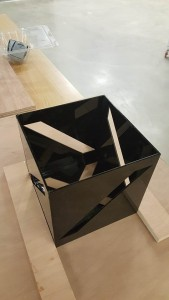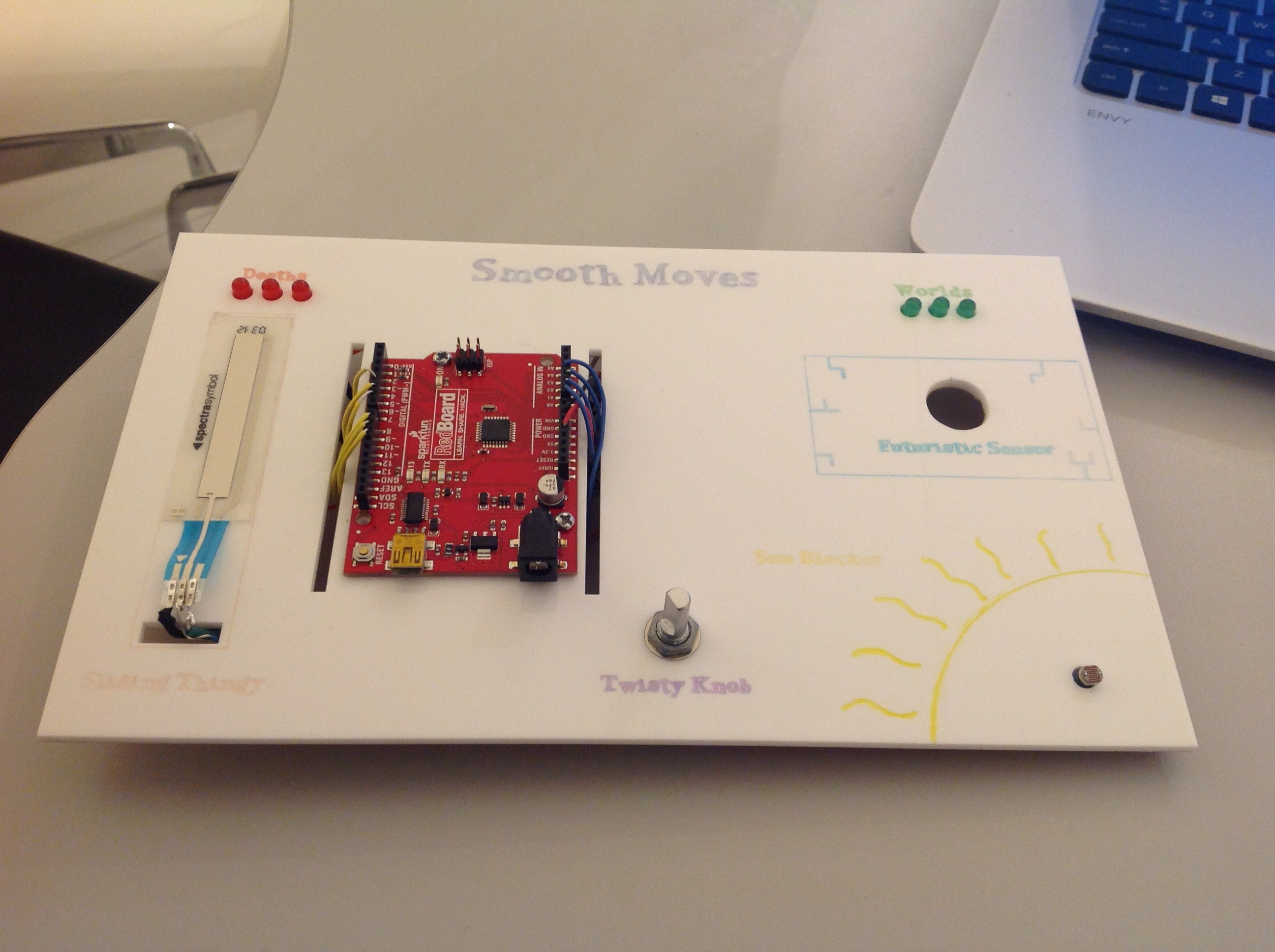## Lamp (Final Project)

For my final project, I have completed making a lamp. The design was hard for me to decide, but through some modification, it ended up like this.## ELLIPSE (the supreme shape)

Discussion of my work process and how I worked out all my designs:

ellispe_documention

```import processing.serial.*;
Serial myPort;
Draw myDraw;
Pulse myPulse;
Random myRandom;
Stretch myStretch;
ArrayList<Sphere> mySphere;
ArrayList<Flow> flows;

boolean ellipse = true;

float values ;
float xPos;
float yPos;

float prevValues = 0;

void setup() {
background(0);
fullScreen(P3D);
//  size(800, 800, P3D);

myDraw = new Draw(width/2,width/2);
myPulse = new Pulse(width/2,height/2);
myRandom = new Random(width/2,height/2);
myStretch = new Stretch();
mySphere = new ArrayList<Sphere>();
flows = new ArrayList<Flow>();
String portName = "/dev/cu.usbserial-DA01LJI0";
myPort = new Serial(this, portName, 9600);
myPort.bufferUntil('\n');
}

void draw() {
noCursor();
println(values,xPos,yPos);
if (values == 0) {
central();
}
if (values == 3) {
ellipse = false;
disco_ball();
}
if (values == 5) {
myDraw.shape_draw();
myDraw.update(xPos,yPos);

ellipse = false;
}
if (values == 7) {
ellipse = false;
myStretch.stretch();
myStretch.update(xPos,yPos);
}
if (values == 9) {
ellipse = false;
myPulse.pulse();
myPulse.update(xPos,yPos);
}
if (values == 11) {
ellipse = false;
myRandom.random_circle();
myRandom.update(xPos,yPos);
}
if (values == 13) {
ellipse = false;
for (int i = flows.size()-1; i>=0; i--) {
Flow s = flows.get(i);
s.update(xPos,yPos);
s.render();
flows.remove(i);
}
}
}
if (values == 15) {
ellipse = false ;
myPulse.pulse();
myPulse.update(xPos,yPos);
}

if (values != prevValues){
clear();
}

prevValues = values;

}

void central() {
pushMatrix();
background(0);
noFill();
stroke(255);
strokeWeight(1.5);
ellipse(width/2, height/2, 300, 300);
popMatrix();
}

void serialEvent(Serial myPort) {

inString = trim(inString);
String[] james = split(inString, ',');

if (james != null) {
values = float(trim(james));
}
//X position
if (james != null) {
james = trim(james);
xPos = float(james);
// println(xPos);
xPos = map(xPos,0,1023,0,width);

}
//Y position
if (james != null) {
james = trim(james);
yPos = float(james);
yPos = map(yPos,1023,0,100,height-100);
}
myPort.write('x');
}

void disco_ball(){
pushMatrix();
background(0);
translate(width/2,height/2,0);
for (int i = mySphere.size()-1; i>=0; i--) { // manipulate the objects
Sphere s = mySphere.get(i);
s.update(xPos,yPos);
s.render();

mySphere.remove(i);
}
}
popMatrix();
}```

```ArrayList<Sphere> spheres;
class Sphere {
float xPos;
float yPos;
float xSpeed;
float ySpeed;
float diam;
float alpha;
float moving_x;
float moving_y;
Sphere() {
alpha = random(127, 350);
xPos = width/4-475;
yPos = height/4-300;
xSpeed = random(-10., 10.);
ySpeed = random(-10., 10.);
diam = 150;
}
if (alpha <= 0) {
return true;
} else {
return false;
}
}
void render() {
strokeWeight(2);
alpha -=3;
diam = diam-3.5;
float yellow = map(diam,100,200,255,0);
float interesting = map(moving_y,0,height,yellow,0);
stroke(255, 255,interesting,alpha);
float cool = map(moving_x,0,width,0,255);
fill(0,cool);
pushMatrix();
translate(xPos,yPos,yPos);
sphere(diam);
popMatrix();
}
void update(float x, float y) {
moving_x = x;
moving_y = y;
xPos+=xSpeed;
yPos+=ySpeed;
}
}```
```class Draw {
int num_vertex;
float centerX;
float centerY;
float Xmove;
float Ymove;
float[] x = new float[num_vertex];
float[] y = new float[num_vertex];

Draw(float _x,float _y) {
Xmove = _x;
Ymove = _y;
smooth();
noFill();
centerX = width/2;
centerY = height/2;
num_vertex = 12;
}
void update(float xPos,float yPos){
Xmove = xPos;
Ymove = yPos;

}
void shape_draw() {
noFill();
stroke(0);
strokeWeight(1);
ellipse(width/2, height/2, 300, 300);
for (int i=0; i<num_vertex; i++) {
x = append(x, cos(angle*i) * radius);
y = append(y, sin(angle*i) * radius);
}
stroke(255, 255, random(0, 250), 80);
if (Xmove != 0 || Ymove != 0) { //WHERE I CHANGED OUT THE MOUSE X AND Y
centerX += (Xmove-centerX) * 0.05;
centerY += (Ymove-centerY) * 0.05;
}

for (int i=0; i<num_vertex; i++) { // cause random movements for verteces within these parameters
x[i] += random(-1.25, 1.25);
y[i] += random(-1.25, 1.25);
}
beginShape();
curveVertex(x[num_vertex-1]+centerX, y[num_vertex-1]+centerY);

for (int i=0; i<num_vertex; i++) { // for every vertex make a point about the center
curveVertex(x[i]+centerX, y[i]+centerY);
}
curveVertex(x+centerX, y+centerY);
curveVertex(x+centerX, y+centerY);
endShape();
}
}```
```class Flow {
// variables for the object
float xPos;
float yPos;
float xSpeed;
float ySpeed;
float alpha;
float size;
float moving_x;
float moving_y;

Flow() {
background(0);
alpha = random(0, 255);
xPos = 0;
yPos = 0;
xSpeed = random(-20,20);
ySpeed = 20;
}

if (alpha <= 0) {
return true;
} else {
return false;
}
}
void update(float new_x, float new_y) {
moving_x = new_x;
moving_y = new_y;
xPos+=xSpeed;
yPos+=ySpeed;
}

void render() {
fill(0);
noStroke();
ellipse(width/2,height/2,300,300);
alpha -=1;
size +=1;
fill(255,255,alpha,alpha);
for(float a = 0; a <=width;a=a+100){
pushMatrix();
float rotationx = map(moving_x,0,width,-PI/8,PI/8);
float rotationy = map(moving_y,0,height,-PI/8,PI/8);
rotateX(rotationx);
rotateY(rotationy);
ellipse(xPos+a,yPos,size,size);
popMatrix();
}
}
}```
```class Pulse {
float parameter_x;
float parameter_y;
float movex;
float movey;

Pulse(float movementsx, float movementsy){
movex = movementsx;
movey = movementsy;
}

void update(float xPos,float yPos){
movex = xPos;
movey = yPos;

}

void pulse(){
if((xPos <970 && xPos>964) &&(yPos<538 && yPos>534)){
parameter_x = random(50,350);
parameter_y = height/2;
}else{
parameter_x = movex;
parameter_y = movey;
if(parameter_x > 350){
parameter_x = 300;
}
}
pushMatrix();
smooth();
frameRate(15);
strokeWeight(2.5);
stroke(255,255,random(0,300));
pushMatrix();
fill(0,20);
rect(-100,-100,width+200,height+200);
popMatrix();
translate(width/2,height/2);
int resolution = (int)map(parameter_y,100,height-100,2, 30);
float angle = TWO_PI/resolution;
beginShape();
for (int i=0; i<=resolution; i++){
float x =  cos(angle*i) * radius;
float y =  sin(angle*i) * radius;
vertex(x, y);
}
endShape();
popMatrix();
}
}```
```class Random{
float x_go;
float y_go;

Random(float movementx,float movementy){
x_go = movementx;
y_go = movementy;
}
void update(float xPos,float yPos){
x_go = xPos;
y_go = yPos;
}

void random_circle(){
smooth();
background(0);
frameRate(45);
pushMatrix();
fill(0,50);
rect(0,0,width,height);
popMatrix();
noStroke();
for (int i=0; i<300; i++){
float circleX = width/2 + cos(angle*i)*150;
float circleY = height/2 + sin(angle*i)*150;
float randomX = random(0,width);
float randomY = random(0,height);

float blue = map(y_go,0,width-50,0,255);
fill(255,255,blue,200);
ellipse(x,y,random(0,20),random(0,20));
}
}
}```
```class Stretch{
float xmove;
float ymove;

void update(float xPos,float yPos ){
xmove = xPos;
ymove = yPos;
}

void stretch(){
background(0);
strokeCap(SQUARE);
smooth();
noFill();
float rotationx = map(xPos,0,width,-PI/6,PI/6);
float rotationy = map(yPos,0,height,-PI/6,PI/6);
rotateX(rotationx);
rotateY(rotationy);

float blue = map(xPos,0,width,0,240);
stroke(255,255,blue,100);

translate(width/2,height/2);
int circleResolution = (int) map(yPos, 0,height, 50,120);
float radius = xPos-width/2 + 50;
float angle = TWO_PI/circleResolution;

strokeWeight(yPos/80+5);

beginShape();
for (int i=0; i<=circleResolution; i++){
float x = cos(angle*i) * radius;
float y = sin(angle*i) * radius;
rect(0,0,x,y);
line(0,0,x,y);
}
endShape();

}

}```

4 of my demos in video:

video-1450330326

## Smooth Moves

I made a fun game titled “Smooth Moves” that makes use of a custom built game controller that consists of several forms of input that I named with wacky names, including: Softpot (Sliding Thingy), a Potentiometer (Twisty Knob), a photocell sensor (Sun Blocker) and a rangefinder/distance sensor (Futuristic Sensor)! Some say it was too hard, ehh n00bs..

Image of final board:## Jumping on the Trampoline!

For the final project, Shelly and I designed a game which players can interact physically with computer program. Our settings are quite simple: a trampoline and a computer. Inspiration for this game came from the offline dinosaur game in google chrome. Players control space key to have dinosaur avoid obstacles when obstacles come one by one. In our game, the basic idea is let players avoid all obstacles. But we pay more attention to players’ interactions with the game. So camera will take player’s head portrait first, after finishing avatar collection, the saved image will be filtered and brought into the game and play the main role. The next step in this game is to control the movement of the main role. The role will meet different obstacles and he must avoid them to keep the game going on by jumping up and down. This movement is not controlled by keys or mouse but plater’s real jump. Player is required to stand on a trampoline and jump, when he jump, his head portrait as a role in the game will jump with him

Scott Jump! https://youtu.be/GklHesXhTTc

## Physical Controller

We learned how to combine arduino and processing together to achieve the real interaction last class. Before it, I only know how to build a circuit to control sensors and code in processing individually. This time, I chose potentiometer as physical controller in my project to control picaman in my picman game. When you touch the different part on the potentiometer, the picmen will move toward different directions.

## Computational Media

My original inspiration to join this class comes from the IM final show in Shanghai. When I saw many amazing works that students made and played with them were super fun. I also want to make something like theirs, so I am so lucky to get into Intro to IM class in Abu Dhabi for my first study away semester. As I came here, everything was new and seems a little bit overwhelming for me. And the first assignment gave me a chance to start to notice and observe interactions in our living environment. Continue reading “Computational Media”